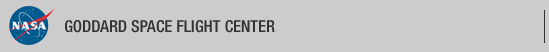+ Visit NASA.gov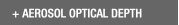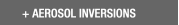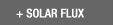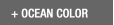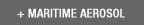Web Site Feature AERONET Data Synergy Tool - Access Earth Science data sets for AERONET sites-Home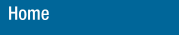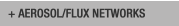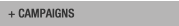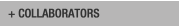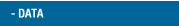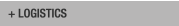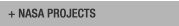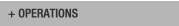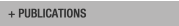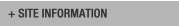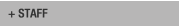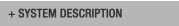AERONET DATA ACCESS

AERONET Site Lists (V3)

+ All ListsDATA - Units

+ Inversion Product Descriptions

Data Products

Direct Sun Measurements
Aerosol Optical Depth (AOD) Levels 1.0, 1.5, and 2.0
AOT_(wavelength)
wavelength in nm
None
%TripletVar is the AOD "triplet variability" multiplied by 100.
%TripletVar_(wavelength)
wavelength in nm
None
Angstrom Parameter
(AOD1)-(AOD2)Angstrom
wavelength range in nm
None
Water Vapor
Water(cm)
wavelength at 940 nm
cm^3/cm^2 or g/cm^2
Spectral Deconvolution Algorithm Output (SDA) from Direct Sun Measurements
Total AOD 500nm
Total_AOD_500nm[tau_a]
wavelength in nm
None
Fine Mode AOD 500nm
Fine_Mode_AOD_500nm[tau_f]
wavelength in nm
None
Coarse Mode AOD 500nm
Coarse_Mode_AOD_500nm[tau_c]
wavelength in nm
None
2nd Order Reg Fit Error - Total AOD 500nm (SDA
2nd_Order_Reg_Fit_Error-Total_AOD_500nm[regression_dtau_a]
wavelength in nm
None
RMSE Fine Mode AOD 500nm
RMSE_Fine_Mode_AOD_500nm[Dtau_f]
wavelength in nm
None
RMSE Coarse Mode AOD 500nm
RMSE_Coarse_Mode_AOD_500nm[Dtau_c]
wavelength in nm
None
RMSE Fine Mode Fraction 500nm
RMSE_FineModeFraction_500nm[Deta]
wavelength in nm
None
Angstrom Exponent - Total 500nm
Angstrom_Exponent(AE)-Total_500nm[alpha]
wavelength in nm
None
dAE/dln(wavelength)-Total 500nm
dAE/dln(wavelength)-Total_500nm[alphap]
wavelength in nm
None
Angstrom Exponent-Fine Mode 500nm
AE-Fine_Mode_500nm[alpha_f]
wavelength in nm
None
dAE/dln(wavelength)-Fine Mode 500nm
dAE/dln(wavelength)-Fine_Mode_500nm[alphap_f]
wavelength in nm
None
Input AOD to SDA
(wavelength)_Input_AOD
wavelength in nm
None
(azimuth angle)
degrees
µW/cm^2/sr/nm

(scattering angle)

from -6 to 6 uses sun collimator

from 6 to 150 uses sky collimator

degrees
µW/cm^2/sr/nm
(zenith angle)P(polarized channel number)
degrees
µW/cm^2/sr/nm
Degree of Polarization
(zenith angle)[degofpol]
degrees
None
Sky and Surface Radiance for BRDF
(scattering angle)(SAZ=(solar azimuth angle)
degrees, degrees
µW/cm^2/sr/nm
zenith
0 degrees
µW/cm^2/sr/nm
Aerosol Inversion Retrievals from Sky Radiance Measurements
center of size bins (logarithmic scale)
µm
10^5 * µm^3/µm^2
Size Distribution
dV(r)/dln r
discrete size points (logarithmic scale)
µm
µm^3/µm^2
Single Scattering Albedo
SSA(wavelength)
wavelength in nm
None
Refractive Index (real part)
REFR(wavelength)
wavelength in nm
None
Refractive Index (imaginary part)
REFI(wavelength)
wavelength in nm
None
Volume Concentration
VolCon
None
µm^3/µm^2
None
µm
None
None
Standard Deviation
StdDev
None
None
AOD Coincident
AOT_(wavelength)
wavelength in nm
None
AOD Extinction
AOTExt(wavelength)
wavelength in nm
None
AOD Absorption
AOTAbsp(wavelength)
wavelength in nm
None
Phase Functions
(scattering angle)[wavelength]
degrees, nm
None
Asymmetry Factor
ASYM(wavelength)
wavelength in nm
None
Downward/Upward at BOA and TOA
wavelength in nm

W/m^2

Spectral Flux
Downward/Upward/Diffuse
wavelength in nm

W/m^2

At BOA and TOA
wavelength in nm

W/m^2

At BOA and TOA
wavelength in nm

W/m^2

Other Data and Parameters
Date/Time
Date, Time
None
GMT Solar Day/GMT Time
N (Number of Measurements for Average)
N[]
None
None
Altitude
Altitude
None
km

Solar Zenith Angle for 1020nm Channel

(average between left and right almucantars)

solar_zenith_angle_for_1020nm _scan
None
Degrees
Average Solar Zenith Angle for Broadband Flux Calculation
average_solar_zenith_angle_for_ flux_calculation
None
Degrees
Julian Day
Julian_Day
None
Days
Size Index, Num of Angles
siz_index,num_of_angles
None
None
Alm_type (Data level identifier)
alm_type
None
None
Sky Error, Sun Error
sky_error
None
%
AOD at 440 nm
tau440(measured)
None
None
Spectral Albedo
Albedo_ Wavelength
wavelength in nm
None
Sphericity Parameter

Sphericity Parameter

None
%
Optical Air Mass
Air_Mass
None
None
Exact Wavelength of Instrument Filter
AOT_(wavelength) Exact Wavelength
wavelength in nm
µm

Key:

cm = centimeter (10E-2 m) ; µm = micrometer (10E-6 m); nm = nanometer (10E-9 m);
µW = microwatt (10E-6 W); mV = millivolts (10E-3 V)

T - Total data mode
C - Coarse data mode
F - Fine data mode+ Privacy Policy and Important Notices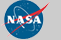Curator: David M. Giles NASA Official: Brent N. Holben Last Updated: July 20, 2021